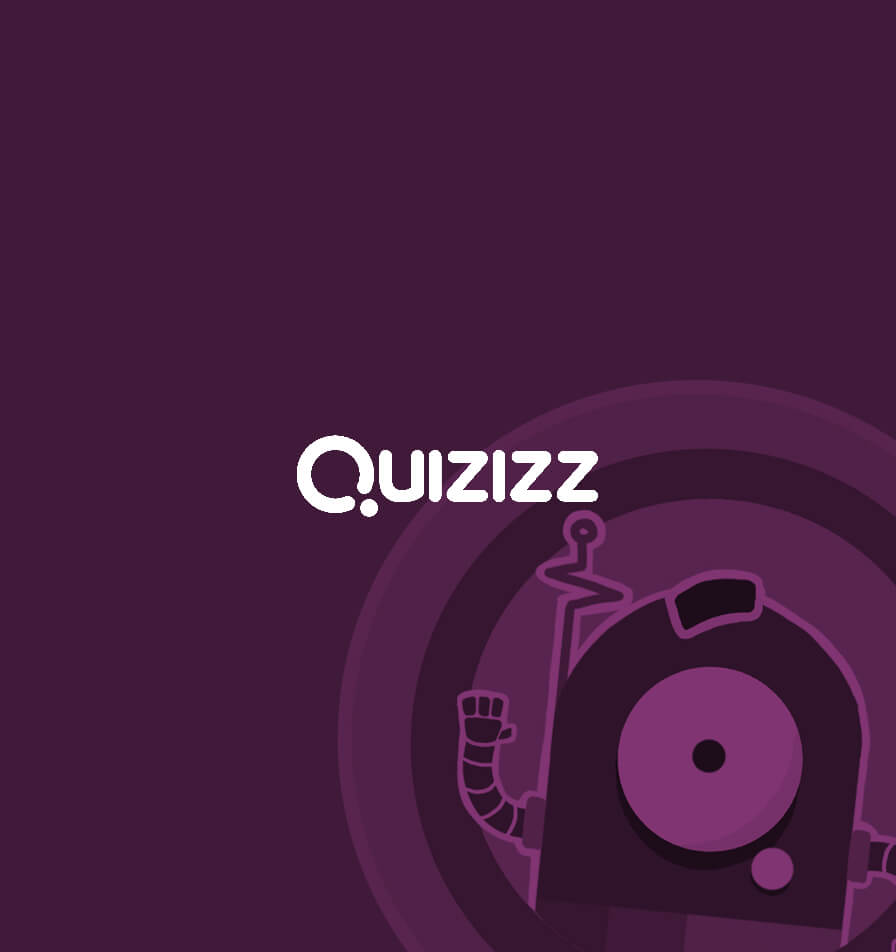LESSONNEW
Unit 6: Probability - Lesson 2
ctout_46310
6 hours ago
Save
Copy and Edit
INSTRUCTOR-LED SESSION
Start a live lesson
ASYNCHRONOUS LEARNING
Assign homework
• Slide 1
Report an issue

Unit 6: Probability

Lesson 2: Probability of Simple Events• Slide 2
Report an issue

Vocabulary

• Probability: The likelihood of an action or event happening during an experiment

• Favorable Outcomes: What we want to happen in an event

• Slide 3
Report an issue

How to calculate probability

• Step 1: List out the Sample Space, and Determine the total number of outcomes

• Step 2: Determine the number of ways our Favorable outcome can occur

• Step 3: Create a Ratio with favorable over total• Question 4
30 seconds
Report an issue
Q.

What is the probability of landing on heads if you toss a coin?

1/2

1/3

1/6

2/2

• Question 5
30 seconds
Report an issue
Q.

What is the probability of rolling a fair number cube and landing on "3"?

1/3

1/2

1/6

1/5

• Slide 6
Report an issue

Probability Scale

• 0% = Impossible to happen

• 25% = Unlikely

• 50% = Even Change

• 75% = Likely

• 100% = Certain• Slide 7
Report an issue

Probability Scale Examples

• The likely hood of rolling a 0 on a number cube is 0% so it is impossible

• The likely hood of getting heads is 50%, so it has an even chance

• The likely hood of rolling a number >0 is 100% so it is certain• Question 8
30 seconds
Report an issue
Q.

What is the Probability of rolling a 4 or P(4)?

1/4

1/5

1/6

1/2

• Question 9
30 seconds
Report an issue
Q.

What is the likely hood of rolling an even number or p(even)?

1/6; unlikely

0/6; impossible

6/6; certain

3/6; Evenly Likely

• Slide 10
Report an issue

• Sometimes you will have probabilities asking for Event 1 or Event 2

• To solve these problems you will find the event probability for each• Question 11
30 seconds
Report an issueQ.

What is the Probability of drawing a green or yellow marble? P(Green OR Yellow)=?

1/2

1/4

1/3

5/12

• Question 12
30 seconds
Report an issueQ.

What is the Probability of drawing a Blue, or Red marble? P(Blue OR Red)=?

1/2

7/12

1/3

5/12

• Slide 13
Report an issue

Complement Events

Are 2 events in which EITHER one or the other MUST happen, but they CANNOT happen at the same time.• Slide 14
Report an issue

How do you know if it is a complement problem?

• When it says: Not [event]

• Ex: What is the probability of Not rolling a number greater than 4?

• Ex: P(Not greater than 4)• Question 15
30 seconds
Report an issueQ.

What is the probability of Not spinning blue?

P(Not Blue) = 1/4

P(Not Blue) = 1/2

P(Not Blue) = 2/3

P(Not Blue) = 3/4

• Question 16
30 seconds
Report an issue
Q.

What is the probability of NOT rolling a Prime Number?

0/6

1/6

1/2

1/3

• Slide 17
Report an issue

Let's Review

Subtitle
• Question 18
30 seconds
Report an issueQ.

What is the probability of drawing D or P(D) = ?

1/4

1/9

1/5

1/3

• Question 19
30 seconds
Report an issueQ.

What is the probability of drawing S, V, or L? P(S,V, or L) = ?

2/3

1/2

1/3

5/9

• Question 20
30 seconds
Report an issueQ.

What is the probability of Not Drawing D? P(Not D) = ?

8/9

7/9

2/3

1/2

• Question 21
30 seconds
Report an issueQ.

This concept scares me